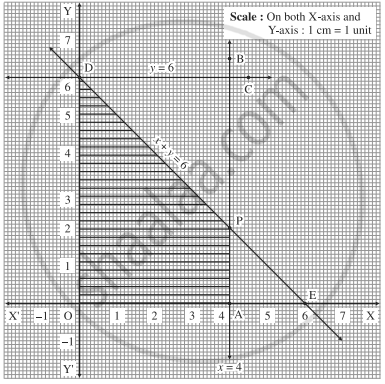Advertisement Remove all ads

# Solve the following LPP by graphical method: Maximize z = 11x + 8y, subject to x ≤ 4, y ≤ 6, x + y ≤ 6, x ≥ 0, y ≥ 0, - Mathematics and Statistics

Graph
Sum

Solve the following LPP by graphical method:

Maximize z = 11x + 8y, subject to x ≤ 4, y ≤ 6, x + y ≤ 6, x ≥ 0, y ≥ 0,

Advertisement Remove all ads

#### Solution

First we draw the lines AB, CD and ED whose equations are x = 4, y = 6 and x + y = 6 respectively.

 Line Equation Points on the X-axis Points on the Y-axis Sign Region AB x = 4 A(4, 0) - ≤ origin side of the line AB CD y = 6 - D(0, 6) ≤ origin side of the line CD EF x + y = 6 E(6, 0) D(0, 6) ≤ origin side of the line EDThe feasible region is shaded portion OAPDO in the graph.

The vertices of the feasible region are O (0, 0), A (4, 0), P and D (0, 6)

P is point of intersection of lines x + y = 6 and x = 4.

Substituting x = 4 in x + y = 6, we get

4 + x = 6    ∴ y = 2          ∴ P is (4, 2)

∴ the corner points of feasible region are O (0, 0), A (4, 0), P (4, 2) and D (0, 6).

The values of the objective function z = 11x + 8y at these vertices are

z(O) = 11(0) + 8(0) = 0 + 0 = 0

z(a) = 11(4) + 8(8) = 44 + 0 = 44

z(P) = 11(4) + 8(2) = 44 + 16 = 60

z(D) = 11(0) + 8(2) = 0 + 16 = 16

∴ z has maximum value 60, when x = 4 and y = 2.

Concept: Linear Programming Problem (L.P.P.)
Is there an error in this question or solution?
Advertisement Remove all ads

#### APPEARS IN

Advertisement Remove all ads
Advertisement Remove all ads
Share
Notifications

View all notifications

Forgot password?